# MDX with Microsoft SQL Server 2008 R2 Analysis Services Cookbook

By Tomislav Piasevoli
• \$30.99 eBook
• \$12.99 eBook + Subscription
What do you get with a Packt Subscription?

• Constantly updated with 100+ new titles each month
• Breadth and depth in over 1,000+ technologies

Microsoft SQL Server is an enterprise database platform that contains a multitude of technologies, Analysis Services being one of them. SQL Server Analysis Services (SSAS) provides OLAP and data mining capabilities and allows users to analyze multidimensional data stored in cubes using the MDX query language.

This cookbook contains over 80 practical, task-based recipes that show how Microsoft SQL Server 2008 R2 Analysis Services solutions can be taken further by enriching them with high-performance MDX calculations and flexible MDX queries.

Packed with immediately usable, real-world recipes, the book starts with elementary techniques that lay the foundation for designing further MDX calculations and queries. Here you will find topics such as iterations on a set, Boolean logic, and dissecting and optimizing MDX calculations.

In the first half of the book you will learn how to efficiently work with time, strings, metadata, calculated members and sets in general, and how to implement MDX solutions that are appropriate in a particular context: a time-aware calculation, a concise report, a calculation relative to another. You will also learn how to implement various types of conditional formatting, how to perform typical MDX calculations like ranks, percentages and averages, and year-to-date calculations.

The book then deep dives into topics such as enhancing cube design with utility dimensions, context-aware calculations, and other advanced topics. In this part you will learn how a utility dimension can be of great help, for example when you want to calculate histograms or implement time-based calculations. The advanced topics also cover parent-child hierchies, recursion, random values, and complex sorts.

Publication date:
August 2011
Publisher
Packt
Pages
480
ISBN
9781849681308

## Chapter 1. Elementary MDX Techniques

In this chapter, we will cover:

• Skipping axis

• Handling division by zero errors

• Setting special format for negative, zero, and null values

• Applying conditional formatting on calculations

• Setting default member of a hierarchy in MDX script

• Implementing NOT IN set logic

• Implementing logical OR on members from different hierarchies

• Iterating on a set in order to reduce it

• Iterating on a set in order to create a new one

• Iterating on a set using recursion

• Dissecting and debugging MDX queries

• Using NON_EMPTY_BEHAVIOR

• Optimizing MDX queries using the NonEmpty() function

• Implementing logical AND on members from the same hierarchy

## Introduction

This chapter presents common MDX tasks and one or more ways to solve them or deal with them appropriately. We'll cover basic principles and approaches such as how to skip an axis and prevent common errors, how to set the default member of a hierarchy, and how to format cell foreground and background colors based on the value in cells.

Then we will tackle the logical operations NOT and OR while leaving the most complex AND logic for the end of the chapter.

The second half of the chapter concentrates on iterations and ways to perform them, followed by optimization of calculations using two of the most common approaches, NonEmpty() function and NON_EMPTY_BEHAVIOR property. Finally, we will cover how to dissect and debug MDX queries and calculations.

Be sure to read the recipes thoroughly.

## Skipping axis

There are situations when we want to display just a list of members and no data associated with them. Naturally, we expect to get that list on rows, so that we can scroll through them nicely. However, the rules of MDX say we can't skip axes. If we want something on rows (which is AXIS(1) by the way), we must use all previous axes as well (columns in this case, which is also known as AXIS(0)).

The reason why we want the list to appear on axis 1 and not axis 0 is because a horizontal list is not as easy to read as a vertical one.

Is there a way to display those members on rows and have nothing on columns? Sure! This recipe shows how.

Follow these steps to set up the environment for this recipe:

1. Start SQL Server Management Studio (SSMS) or any other application you use for writing and executing MDX queries and connect to your SQL Server Analysis Services (SSAS) 2008 R2 instance (`localhost` or `servername\instancename`).

2. Click on the New Query button and check that the target database is Adventure Works DW 2008R2.

### How to do it…

Follow these steps to get a one-dimensional query result with members on rows:

1. Put an empty set on columns (AXIS(0)). Notation for empty set is this: `{}`.

2. Put some hierarchy on rows (AXIS(1)). In this case we used the largest hierarchy available in this cube – Customer hierarchy of the same dimension.

3. Run the following query:

```SELECT
{ } ON 0,
{ [Customer].[Customer].[Customer].MEMBERS } ON 1
FROM
```

### How it works…

Although we can't skip axes, we are allowed to provide an empty set on them. This trick allows us to get what we need – nothing on columns and a set of members on rows.

### There's more…

Notice that this type of query is very convenient for parameter selection of another query as well as for search. See how it can be modified to include only those customers whose name contains the phrase "John":

```SELECT
{ } ON 0,
{ Filter(
[Customer].[Customer].[Customer].MEMBERS,
InStr(
[Customer].[Customer].CurrentMember.Name,
'John'
) > 0
)
} ON 1
FROM

In the final result, you will notice the "John" phrase in various positions in member names: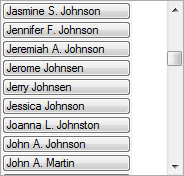#### The idea behind

If you put a cube measure or a calculated measure with a non-constant expression on axis 0 instead, you'll slow down the query. Sometimes it won't be so obvious, sometimes it will. It will depend on the measure's definition and the number of members in the hierarchy being displayed. For example, if you put the `Sales Amount` measure on columns, that measure will have to be evaluated for each member in the rows. Do we need those values? No, we don't. The only thing we need is a list of members; hence we've used an empty set. That way, the SSAS engine doesn't have to go into cube space. It can reside in dimension space which is much smaller and the query is therefore more efficient.

#### Possible workarounds

In case of a third-party application or a control which has problems with this kind of MDX statement (i.e. expects something on columns and is not working with an empty set), we can define a constant measure (a measure returning null, 0, 1 or any other constant) and place it on columns instead of that empty set. For example, we can define a calculated measure in the MDX script whose definition is 1, or any other constant value, and use that measure on the columns axis. It might not be as efficient as an empty set, but it is a much better solution than the one with a regular (non-constant) cube measure like the `Sales Amount` measure.

## Handling division by zero errors

Another common task is handling errors, especially `division by zero` type of errors. This recipe offers a way to solve that problem.

### Note

Not all versions of Adventure Works database have the same date range. If you're not using the recommended version of it, the one for the SSAS 2008 R2, you might have problems with queries in this book. Older versions of Adventure Works database have dates up to the year 2006 or even 2004. If that's the case, make sure you adjust examples by offsetting years in the query with a fixed number. For example, the year 2006 should become 2002 and so on.

Start a new query in SQL Server Management Studio and check that you're working on Adventure Works database. Then write and execute this query:

```WITH
MEMBER [Date].[Calendar Year].[CY 2006 vs 2005 Bad] AS
[Date].[Calendar Year].[Calendar Year].& /
[Date].[Calendar Year].[Calendar Year].&,
FORMAT_STRING = 'Percent'
SELECT
{ [Date].[Calendar Year].[Calendar Year].&,
[Date].[Calendar Year].[Calendar Year].&,
[Date].[Calendar Year].[CY 2006 vs 2005 Bad] } *
[Measures].[Reseller Sales Amount] ON 0,
{ [Sales Territory].[Sales Territory].[Country].MEMBERS }
ON 1
FROM

This query returns 6 rows with countries and 3 rows with years, the third row being the ratio of the previous two, as its definition says.

The problem is that we get 1.#INF on some cells. To be precise, that value (the formatted value of infinity), appears on rows where the CY 2005 is null. Here's a solution for that.

### How to do it…

Follow these steps to handle division by zero errors:

1. Copy the calculated member and paste it as another calculated member. During that, replace the term `Bad` with `Good` in its name, just to differentiate those two members.

2. Copy the denominator.

3. Wrap the expression in an outer `IIF()` statement.

4. Paste the denominator in the condition part of the `IIF()` statement and compare it against 0.

5. Provide null value for the True part.

6. Your initial expression should be in the False part.

7. Don't forget to include the new member on columns and execute the query:

```MEMBER [Date].[Calendar Year].[CY 2006 vs 2005 Good] AS
IIF ([Date].[Calendar Year].[Calendar Year].& = 0,
null,
[Date].[Calendar Year].[Calendar Year].& /
[Date].[Calendar Year].[Calendar Year].&
),
FORMAT_STRING = 'Percent'
```
8. The result shows that the new calculated measure corrects the problem – we don't get errors (the rightmost column, compared to the one on its left):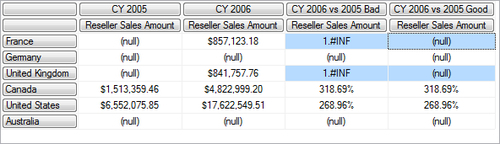### How it works…

A division by zero error occurs when the denominator is null or zero and the numerator is not null. In order to prevent this error, we must test the denominator before the division and handle the case when it is null or zero. That is done using an outer IIF() statement.

It is enough to test just for zero because `null = 0` returns True.

### There's more…

SQLCAT's SQL Server 2008 Analysis Services Performance Guide has lots of interesting details regarding the IIF() function: http://tinyurl.com/PerfGuide2008

Additionally, you may find Jeffrey Wang's blog article useful in explaining the details of the IIF() function: http://tinyurl.com/IIFJeffrey

#### Earlier versions of SSAS

If you're using a version of SSAS prior to 2008 (that is, 2005), the performance will not be as good. See Mosha Pasumansky's article for more info: http://tinyurl.com/IIFMosha

## Setting special format for negative, zero and null values

The FORMAT_STRING property is used for specifying how a regular or calculated member (usually a measure) should be presented and what its FORMATTED_VALUE should look like. There are some predefined formats such as Standard, Currency or Percent, but we can also specify our own using the right combination of characters.

Most people are unaware of the fact that there are actually 4 sections of the format string for numeric values and 2 sections for strings. In this recipe we'll see how to set the format for negative, zero, or null values.

Follow these steps to set up the environment for this recipe:

1. Open Business Intelligence Development Studio (BIDS) and then open the Adventure Works DW 2008 solution.

2. Double-click the Adventure Works cube and go to the Calculations tab.

3. Choose Script View.

4. Position the cursor at the end of the script and create a new calculated measure as follows:

```Create Member CurrentCube.[Measures].[Gross Profit formatted]
As [Measures].[Sales Amount] -
[Measures].[Total Product Cost],
Associated_Measure_Group = 'Sales Summary';```

That's actually the same definition as the definition of the `Gross Profit` measure. We have created a clone of that measure using a different name so that we can compare them later and see the formatting effect in action.

### How to do it…

Follow these steps to set a custom format:

1. Add the `Format_String` property for that measure.

2. Be sure to specify it as below, using 4 sections separated by a semi-colon (;):

```Create Member CurrentCube.[Measures].[Gross Profit formatted]
As [Measures].[Sales Amount] -
[Measures].[Total Product Cost],

Format_String = "#,##0;- #,##0;0;n/a",

Associated_Measure_Group = 'Sales Summary';```
3. Save and deploy (or just press the Deploy MDX Script icon if you're using BIDS Helper).

4. Go to the Cube Browser tab. Reconnect and click on the Show Empty Cells button.

5. Put Promotion Category level on rows. Put Gross Profit and the new Gross Profit formatted measures on columns. Both measures have the same values, but they are formatted differently, as shown in the following image: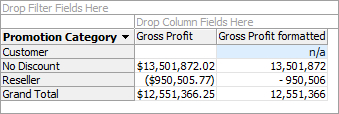6. Notice that the new measure shows n/a for null values and uses minus sign for negative ones. In addition to that, it removes decimal part of numbers.

### How it works…

The four sections of the format string for numeric values are as follows: the first is for positive numbers, the second is for negative numbers, the third is for the zero value, and the fourth is for null values.

Usually we provide a format string for one section only. However, when required, we can use additional sections and provide formatting there as well. In the example, we eliminated decimals from the value, emphasized negative values with a space before a minus sign, and provided n/a for null values.

### There's more…

Another way of specifying format string is by using the `Format_String()` function inside the MDX script:

```Format_String( [Measures].[Gross Profit formatted] ) =
"#,##0;;;-";```

By the way, the Adventure Works DW 2008R2 database has a similar example at the end of the MDX script.

We can omit the format of one section by closing it with a semicolon. In the expression above, the format has been specified for positive and null values only. The negative and zero values will retain their default format.

In fact, the format string can be set for several measures that way.

```Format_String( { [Measures].[Gross Profit],
[Measures].[Gross Profit formatted] } ) =
"#,##0;;;-";```

Finally, the set of members doesn't have to be explicit. It can be obtained using MDX calculations. That might be a nice way to handle and maintain formats for all measures in a cube if there's an order with prefixes/suffixes/special characters inside their names. For example, this code shows how to search for a specific pattern in measures names and apply an appropriate format in case of a hit.

```Format_String( Filter( Measures.ALLMEMBERS,
InStr( Measures.CurrentMember.Name,
'Ratio' ) > 0 )
) = 'Percent';

Format_String( Filter( Measures.ALLMEMBERS,
InStr( Measures.CurrentMember.Name,
'Amount' ) > 0 )
) = '#,##0.00';```

#### Tips and tricks

MDX expressions can be used inside the `FORMAT_STRING` property. The calculation should return a string value which will then be used as a format string. Note that only the values of cells are cached by Analysis Services. When complex expressions are used for the `FORMAT_STRING` property, it may improve performance if you wrap that expression in a calculated measure. You can then use the calculated measure in the assignment to the `FORMAT_STRING` property.

#### A friendly warning

Remember never to put zero values inside your cube instead of empty values or your measure will become dense, always returning a non-null value and the performance will suffer because many MDX functions will not be able to operate in bulk mode by taking advantage of the sparsity of the measure.

If it is required that null values are shown as zeros, then leave them as nulls and use the format string instead. Setting the NullHandling property to Preserve on the measure's source column preserves the null value instead of converting it to zero.

#### Troubleshooting formatted values

By default, a query with no `CELL PROPERTIES` returns `VALUE` and `FORMATTED_VALUE`, which means the data is returned in two shapes – raw and formatted. And such are almost all queries. However, if we explicitly omit the `FORMATTED_VALUE` property from cell properties, we won't have formatted values. The success of this recipe depends therefore on the SSAS front end you're using and its way of displaying values.

In case of a problem, be sure to check whether there's an option in the program's connection or its display properties which can turn this on or help in another way. Sometimes you'll be lucky, other times you won't. For example, only the first three sections work in Excel 2007 and Excel 2010. The fourth section is simply ignored.

You can find more information about it on MSDN, on the Using Cell Properties (MDX) page: http://tinyurl.com/CellProperties

#### Formatting options in detail

There are various options for using format strings but we cannot cover them all in this book. If you require additional information regarding this topic, follow these links:

The next recipe, Applying conditional formatting on calculations, shows how to additionally emphasize data by using properties such as `BACK_COLOR` and `FORE_COLOR`.

## Applying conditional formatting on calculations

Sometimes we want to emphasize cells based on their values. That way data analysis becomes easier because we combine best of charts and tables – we still see the values while we have additional color information about the value in each cell.

A typical case is highlighting the background of cells with green or red color for good or bad results, respectively. It's a pretty trivial operation in Excel and it's not that hard in MDX either. This recipe demonstrates how to do this.

Open BIDS and verify you have the Gross Profit formatted measure defined in the previous recipe, Setting special format for negative, zero and null values. If not, define the measure like this:

```Create Member CurrentCube.[Measures].[Gross Profit formatted]
As [Measures].[Sales Amount] -
[Measures].[Total Product Cost],
Format_String = "#,##0;;;-",
Associated_Measure_Group = 'Sales Summary';```

### How to do it…

Follow these steps to apply conditional formatting:

1. Locate the measure's definition and position of the cursor at the beginning of any line between the definition and the end of the measure.

```Fore_Color = RGB(255, 255, 255),
Back_Color = case
when [Measures].CurrentMember > 0
then RGB(0, 128, 0)
when [Measures].CurrentMember < 0
then RGB(128, 0, 0)
else  RGB(100, 100, 100)
end,```
3. Save and deploy (or just press Deploy MDX Script icon if you're using BIDS Helper).

4. Go to Cube Browser tab. Reconnect and click on the Show Empty Cells button.

5. Put the Promotion attribute hierarchy on rows. Put Gross Profit and the new Gross Profit formatted measures on columns. Notice the effect of the format string in the following image. Positive values are colored green, negative ones have become maroon and everything else is grey.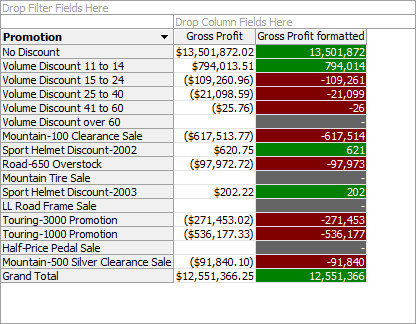### How it works…

The `FORE_COLOR` property can be applied to any calculated member, the same as the `BACK_COLOR` property. Just like with the `FORMAT_STRING` property, we can provide a constant value or an expression. If we provide the expression, the color varies based on what we specified in that expression. If it's a constant, the color remains on all the cells.

In this example, we specified a green background (lighter shade) for positive values, dark red for negative values (dark shades) and grey for the rest (zero and null). Since the background became dark, we changed the font color (or foreground) to white, to keep the contrast high.

The value to be assigned is a number derived by multiplying the factors of 3 colors, components and their values. RGB function found in VBA assembly comes to the rescue here, so we don't have to do that math. That assembly is available by default and registered on every SSAS server.

The arguments of RGB() function are values of intensity of red, green and blue color, in that order. Higher values represent lighter colors, lower values represent darker ones. For example, the black color is (0, 0, 0), whereas white is (255, 255, 255). Everything in between is given to you to experiment with. Take some time and try it!

### There's more…

The foreground and background colors can be changed for regular cube measures as well. Since that property is not directly exposed in the GUI, we have to use the `Fore_Color()` and `Back_Color()` functions in MDX script. Here's one example:

```Back_Color( [Measures].[Order Quantity] ) =
RGB(200, 200, 100);```

#### Tips and tricks

There's a color picker in the Calculation tab of the cube editor. When you operate in the Form View, two color pickers are available, one for the Fore color property of the measure and the other for the Back color property of the measure. Both are found under the Color Expressions section.

When you're using the Script View, the color picker is available in the toolbar.

The color picker returns a single value for a particular color but it also specifies the RGB values in the comment. You may find using the RGB values more convenient. If so, simply delete the single value and apply the RGB() function on RGB values instead.

Advanced graphical programs have color-picker features which lets you point to any dot on the screen and pick its color. As before, RGB values can be read and used in the calculations. This saves you from having to find a particular color if that color is already available somewhere, that is in the company brochure, an Excel table, chart, and so on.

Finally, here are a few sites to help you with colors and their names:

500+ Named Colours with rgb and hex values:

http://tinyurl.com/RGBColors1

RGB to Color Name Mapping:

http://tinyurl.com/RGBColors2

#### Warning

Cells have various properties like value, font, color, etc., but only some of them are returned by default, to make the result more compact and faster: `VALUE`, `FORMATTED_VALUE` and `CELL_ORDINAL`. You can verify this by double-clicking on any cell with the value in the result of an MDX query in SQL Server Management Studio.

Other properties, `FORE_COLOR` and `BACK_COLOR`, can be retrieved on demand, using the `CELL PROPERTIES` MDX keyword, an optional part of the query. When that keyword is used, only the explicitly stated properties are returned. In other words, if we want to include the color properties, we should specify those default properties explicitly or they won't be included in the result.

The success of this recipe ultimately depends on the SSAS front end you're using and its way of displaying values. It may be that in its default mode the front end omits cell properties in order to display results faster. If so, be sure to check whether there's an option in the program's connection or display properties which can turn them on when requested. If not, see if you can edit the MDX and insert the `FORE_COLOR` and the `BACK_COLOR` keywords in the `CELL PROPERTIES` part of the query. If that part is not there at all, you must add it at the end of the query by specifying the `CELL PROPERTIES` keyword and then enlisting all the properties you want to be returned. The complete list of cell properties can be found here: http://tinyurl.com/CellProperties

The Recipe entitled Setting special format for negative, zero and null values shows how to provide more than one format based on the value of the calculation using the `FORMAT_STRING` property and its sections.

## Setting default member of a hierarchy in MDX script

Setting a default member is a tempting option which looks like it can be used on any dimension we would like. The truth is far from that. Default members should be used as exceptions and not as a general rule when designing dimensions.

The reason for that is not so obvious. The feature looks self-explanatory and it's hard to anticipate what could go wrong. If we're not careful enough, our calculations can become unpredictable, especially on complex dimensions with many relations among attributes.

Default members can be defined in three places. The easy-to-find option is the dimension itself, using the DefaultMember property found on every attribute. The second option is the role, on Dimension Data tab. Finally, default members can be defined in MDX script. One of the main benefits of this place is easy maintenance of all default members in the cube because everything is in one place and in the form of an easy-to-read text. That is also the only way to define the default member of a role-playing dimension.

In this recipe we'll show the most common option, that is, the last one or how to set a default member of a hierarchy in MDX script. More information on setting the DefaultMember is available here:

http://tinyurl.com/DefaultMember

Follow these steps to set up the environment for this recipe:

1. Start SSMS and connect to your SSAS 2008 R2 instance.

2. Click on the New Query button and check that the target database is Adventure Works DW 2008R2. Then execute the following query:

```WITH
MEMBER [Measures].[Default account] AS
[Account].[Accounts].DefaultMember.Name
SELECT
{ [Measures].[Amount],
[Measures].[Default account] } ON 0
FROM
3. The results will show that the default member is Net Income account and its value in this context is a bit more than 12.6 million USD.

4. Next, open Adventure Works DW 2008 solution in BIDS.

5. Double-click on the Adventure Works cube and go to the Calculations tab. Choose Script View.

6. Position the cursor at the beginning of the script, just below the `CALCULATE` command.

### How to do it…

Follow these steps to set a new default member:

1. Enter the following expression to set a new default account:

```ALTER CUBE CurrentCube
UPDATE DIMENSION [Account].[Accounts],
Default_Member = [Account].[Accounts].&;
//Operating Profit```
2. Save and deploy (or just press the Deploy MDX Script icon if you're using BIDS Helper).

3. Run the previous query again.

4. Notice the result has changed. The new default account is Operating Profit, the one we specified in the MDX script using `ALTER CUBE` command. The value changed as well – now it's above 16.7 million USD.

### How it works…

The `ALTER CUBE` statement changes the default member of a hierarchy specified in the `UPDATE DIMENSION` part of the statement. The third part is where we specify which member should be the default member of that hierarchy.

Don't mind that it says "UPDATE DIMENSION". SSAS 2005 and above interpret that as hierarchy.

### There's more…

Setting the default member on a dimension with multiple hierarchies can lead to unexpected results. Due to attribute relations, related attributes are implicitly set to corresponding members while the non-related attributes remain on their default members, that is, the All member (also known as the root member). Certain combinations of members from all available hierarchies can result in a non-existing coordinate. In that case, the query will return no data. Other times, the intersection will only be partial. In that case, the query will return the data, but the values will not be correct which might be even worse than no data at all.

Here's an example illustrating that:

Enter the following expression in the MDX script, deploy it, and then analyze the result in the Cube Browser tab.

```ALTER CUBE CurrentCube
UPDATE DIMENSION [Date].[Calendar],
Default_Member = [Date].[Calendar]
.[Calendar Year].&;
-- "current" year on the user hierarchy```

The expression sets the year 2007 as the default member of the `[Date].[Calendar]` user hierarchy.

The analysis of the Sales Amount measure in the Cube Browser shows good results in almost all cases except in few. Fiscal hierarchies that have the fiscal year level in them return empty or incomplete results when used in slicer. Empty, because the intersection between the fiscal year 2006 and the calendar year 2007 (the latter being the default member in the calendar hierarchy) is a non-existing combination. Remember, the calendar year 2007 doesn't get overwritten by the fiscal 2006. It gets combined (open the Date dimension in BIDS and observe the relations in the corresponding tab). Moreover, when you put the fiscal year 2007 in slicer, you only get a portion of data, the portion which matches the intersection of the calendar and the fiscal year. That's only one half of the fiscal year, right? In short, you have a potential problem with this approach.

Can we fix the result? Yes, we can. The correct results will be there when we explicitly select the All member from the Date.Calendar hierarchy in the slicer. Only then we get good results using fiscal hierarchies. The question is – will the end-users remember that every time?

The situation is similar when the default member is defined on an attribute hierarchy, for example, on the Date.Calendar Year hierarchy. By now, you should be able to modify the previous expression so that it sets the year 2007 as the default member on the `[Date].[Calendar Year]`. Test this to see it for yourself.

Another scenario could be that you want to put the current date as the default member on the Date.Date hierarchy. Try that too, and see that when you use the year 2006 from the Date.Calendar Year hierarchy in slicer, you get an empty result. Again, the intersection formed a non-existing coordinate.

To conclude, you should avoid defining default members on complex dimensions. Define them where it is appropriate: on dimensions with a single non-aggregatable attribute (that is when you set the IsAggregatable property of an attribute to False) or on dimensions with one or more user hierarchies where that non-aggregatable attribute is the top level on each user hierarchy and where all relations are well made.

The Account dimension used in this example is not such a dimension. In order to correct it, two visible attributes should be made hidden because they can cause empty results when used in slicer. Experimenting with scope might help too, but that adds to the complexity of the solution and hence the initial advice of keeping things simple when using default members should prevail.

Take a look at other dimensions in the Adventure Works DW 2008 database. There you will find good examples of using default members.

When you're defining the default members in MDX script, do it in the beginning of the script. This way the calculations that follow can reference them.

In addition, provide a comment explaining which member was chosen to be the default member and optionally why. Look back at the code in this recipe to see how it was done.

## Implementing NOT IN set logic

There are times when we want to exclude some members from the result. We can perform this operation using a set of members on an axis or using a set of members in slicer, that is, the `WHERE` part of an MDX query. This recipe shows how to do the latter, how to exclude some members from a set in slicer. The principle is the same for any part of an MDX query.

Start a new query in SSMS and check that you're working on the right database. Then type in the following query and execute it:

```SELECT
{ [Measures].[Reseller Order Count] } ON 0,
NON EMPTY
{ [Promotion].[Promotion].MEMBERS }
DIMENSION PROPERTIES
[Promotion].[Promotion].[Discount Percent]
ON 1
FROM

The above query returns 12 promotions. The DIMENSION PROPERTIES keyword is used to get additional information about members – their discount percent. That property can be seen among other properties by double-clicking each member on rows.

Our task is to exclude promotions with a discount percent of 0, 2 and 5.

### How to do it…

Follow these steps to reverse the set:

1. Navigate to the Promotion dimension and expand it.

2. Expand the Discount Percent hierarchy and its level.

3. Take the first 3 members (with the names 0, 2 and 5) and drag them one by one below the query, then form a set of them using curly brackets.

4. Expand the query by adding the `WHERE` part.

5. Add the set with those 3 members using a minus sign in front of the set.

```SELECT
{ [Measures].[Reseller Order Count] } ON 0,
NON EMPTY
{ [Promotion].[Promotion].MEMBERS }
DIMENSION PROPERTIES
[Promotion].[Promotion].[Discount Percent]
ON 1
FROM
WHERE
( - { [Promotion].[Discount Percent].&,
[Promotion].[Discount Percent].&[2.E-2],
[Promotion].[Discount Percent].&[5.E-2] } )```
6. Execute the query and see how the results change. Double-click each promotion and verify that no promotion has discount percent equal to 0, 2 or 5 anymore.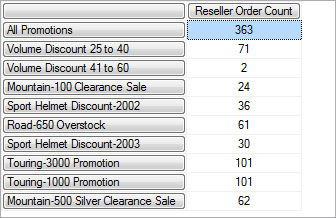### How it works…

The initial query is not sliced by discount percentages. We can think of it as if all the members of that hierarchy are being there in the slicer:

```WHERE ( { [Promotion].[Discount Percent]
.[Discount Percent].MEMBERS } )```

Of course, we don't have to write such expressions; the SSAS engine takes care of it by default. In other words, we're fine until the moment we want to change the slicer by either isolating or removing some members from that set. That's when we have to use that hierarchy in slicer.

Isolation of members is simply done by enumerating them in slicer. Reduction, the opposite operation, is performed using the `Except()` function:

```WHERE ( Except( { [Promotion].[Discount Percent]
.[Discount Percent].MEMBERS },
{ [Promotion].[Discount Percent].&,
[Promotion].[Discount Percent].&[2.E-2],
[Promotion].[Discount Percent].&[5.E-2] }
)
)```

The alternative for the `Except()` function is a minus sign, which brings us to the shorter version of the previous expression, the version that was used in this recipe.

When a minus sign is used between two sets, it performs the same difference operation between those sets as `Extract()` does. When the first set is missing, set of all members is implicitly added as the first set. The difference between all members and the members of any set is the opposite set of that set. This is how you can perform the NOT IN logic on sets. Both variants work, but the one with the minus sign in front of the set is hopefully easier to remember.

### There's more…

If we open the Promotion dimension inside BIDS, we'll notice that the Discount Percent attribute has the `MemberValue` property defined. The value of that property is equal to a discount percentage and therefore in this particular case we could write an equivalent syntax:

```WHERE
( { Filter( [Promotion].[Discount Percent]
.[Discount Percent].MEMBERS,
[Promotion].[Discount Percent]
.CurrentMember.MemberValue >= 0.1 ) } )```

The advantage of this expression is that it should filter out additional members with a percentage less than 10% if they ever appear on that hierarchy. If we're not expecting such a case or if we strictly want to exclude certain, not necessarily consecutive, members from the hierarchy (Unknown Member, NA member, and so on), we should use the first example: the one with explicit members in the set.

The next recipe, Implementing logical OR on members from different hierarchies is based on a similar theme as this recipe.

## Implementing logical OR on members from different hierarchies

The nature of a multidimensional database and its underlying structures has a direct consequence on how we should write combinations of members. Some combinations are there by design, others require a bit of imagination.

For example, a set of two members of the same hierarchy (colors black and white) placed in a slicer automatically applies OR logic on the result. This means that the result will have data where the first, the second or both of the members (or at least one of their descendants to be precise) occurred in the underlying fact table. In other words, where the product sold was either black or white. The emphasis is on two things: the set and the OR word. In other words, OR logic manifests in sets.

The other example is a tuple formed by two members from different hierarchies (i.e. color black and size XL). Once placed in slicer, this tuple guarantees that the resulting rows will have data on that exact slice, meaning, on both members (or at least one of the descendants of each to be precise). Here, the emphasis is again on two things: the tuple and the AND word. In other words, AND logic manifests in tuples.

Let's summarize. In MDX, a set is by default the equivalent of logical OR while a tuple is by default the equivalent of logical AND. So where's the problem?

The problem is we can only put members of different hierarchies in a tuple and of the same hierarchy in a set. Which means we're missing two combinations, different hierarchies using OR and the same hierarchy using AND.

This recipe shows how to implement OR logic using members from different hierarchies. The last recipe in this chapter shows how to perform AND logic using members from the same hierarchy. It is recommended that you read both recipes.

Start a new query in SSMS and check that you're working on the right database. Then type in the following query and execute it:

```SELECT
{ [Measures].[Reseller Order Quantity],
[Measures].[Reseller Order Count] } ON 0,
NON EMPTY
{ [Product].[Subcategory].MEMBERS } ON 1
FROM
WHERE
( [Product].[Color].&[Black] )```

The query displays 10 product subcategories containing black products.

Next, open a new query window and execute the following query.

```SELECT
{ [Measures].[Reseller Order Quantity],
[Measures].[Reseller Order Count] } ON 0,
NON EMPTY
{ [Product].[Subcategory].MEMBERS } ON 1
FROM
WHERE
( [Product].[Size Range].&[XL] )```

It's a query similar to the previous one, but this one returns only product subcategories containing XL size range products. There's only one product subcategory in the result - Jersey.

The task is to combine these queries so that we get the result of OR operation on those two conditions, in a single query, of course.

### How to do it…

Follow these steps to apply the OR logic with members from different hierarchies:

1. Copy-paste the first query in the new window.

2. Copy-paste the member in slicer from the second query and put it in slicer as well.

3. Notice that those two members originate from different hierarchies and that we cannot put them in a set.

4. Wrap each member in brackets so that they become tuples.

5. Put a comma between those two tuples and wrap them in curly brackets so that they form a multidimensional set of two tuples.

6. Add the root member of the opposite hierarchy in each tuple while preserving the order of hierarchies in them.

7. You're done. The query should look as follows. Notice how the color and size range hierarchies have the same order in those tuples. That's the way it should be.

```SELECT
{ [Measures].[Reseller Order Quantity],
[Measures].[Reseller Order Count] } ON 0,
NON EMPTY
{ [Product].[Subcategory].MEMBERS } ON 1
FROM
WHERE
(
{ ( [Product].[Color].&[Black],
,
[Product].[Size Range].&[XL] ) }
)```
8. Next, execute that query and check that you have 12 rows including the total on top: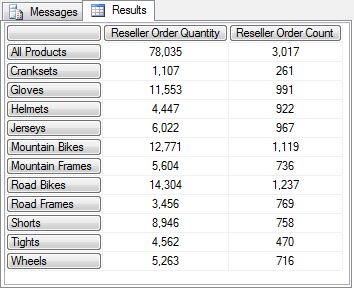### How it works…

Logical OR represents a set. Since we have members of different dimensionality, we must first convert them to tuples of the same dimensionality. That is done by expanding each with the other one's root member and enclosing the expression in brackets (which is how we convert a member to a tuple). Once we have compatible tuples, we can convert them into a set by separating them with a comma and adding curly brackets around the whole expression. This is the standard way that we enumerate members in single-dimensional sets. Multi-dimensional sets are no different except it's the tuples that we're enumerating this time.

### There's more…

We can also use the `UNION()` function instead of enumerating members in the set. The union has an extra feature, an option to remove or preserve duplicates in the resulting set. While that feature is of little interest when the slicer is concerned, it might be interesting when the same logic is applied in calculations. Keep that in mind.

#### A special case of a non-aggregatable dimension

In case your dimension has no root member (eliminated by setting the property IsAggregatable to False) use its default member instead.

#### A very complex scenario

In this recipe we used two hierarchies of the same dimension because this is often the case in real life. However, that's not a limitation. The solution is applicable to any dimension and its hierarchies. For example, when you need to combine 3 different hierarchies you can apply the same solution, thereby expanding each member into tuple with N-1 root members (here N=2) and creating a set of N such tuples.

In case you need to combine many members using OR logic, sometimes with even more than one of them on the same hierarchy and others on different hierarchies, you need to apply the knowledge about dimensionality – members of the same hierarchy should be enlisted in a set, members of different dimensions should be combined with root members of other hierarchies. You just need to be careful with various brackets. The `AsymmetricSet()` function from the Analysis Services Stored Procedure Project may help construct complex sets: http://tinyurl.com/AsymmetricSet

The Implementing NOT IN set operation recipe is based on a similar theme to this recipe.

For more information on default members, take a look at the Setting default member of a hierarchy in MDX script recipe.

## Iterating on a set in order to reduce it

Iteration is a very natural way of thinking for us humans. We set a starting point, we step into a loop, and we end when a condition is met. While we're looping, we can do whatever we want: check, take, leave, and modify items in that set. Being able to break down the problems in steps makes us feel that we have things under control. However, by breaking down the problem, the query performance often breaks down as well. Therefore, we have to be extra careful with iterations when data is concerned.

If there's a way to manipulate the collection of members as one item, one set, without cutting that set into small pieces and iterating on individual members, we should use it. It's not always easy to find that way, but we should at least try.

This and the next two recipes show how to perform iteration. They deal with those cases when there's no other way but to iterate. However, some of the recipes also point out which calculation patterns we must recognize and thereby give up on using classic naïve iteration and use a better approach.

Start a new query in SSMS and check that you're working on the right database. Then write the following query:

```SELECT
{ [Measures].[Customer Count],
[Measures].[Growth in Customer Base] } ON 0,
NON EMPTY
{ [Date].[Fiscal].[Month].MEMBERS } ON 1
FROM
WHERE
( [Product].[Product Categories].[Subcategory].& )```

The query returns fiscal months on rows and two measures: a count of customers and their growth compared to the previous month. Mountain bikes are in slicer.

Now let's see how we can get the number of days the growth was positive for each period.

### How to do it…

Follow these steps to reduce the initial set:

1. Create a new calculated measure in the query and name it `Positive growth days`.

2. Specify that you need descendants of current member on leaves.

3. Wrap around the `FILTER()` function and specify the condition which says that the growth measure should be greater than zero.

4. Apply the `COUNT()` function on a complete expression to get count of days.

5. The new calculated member's definition should look as follows, verify that it does.

```WITH
MEMBER [Measures].[Positive growth days] AS
FILTER(
DESCENDANTS([Date].[Fiscal].CurrentMember, , leaves),
[Measures].[Growth in Customer Base] > 0
).COUNT```
6. Add the measure on columns.

7. Run the query and observe if the results match the following image: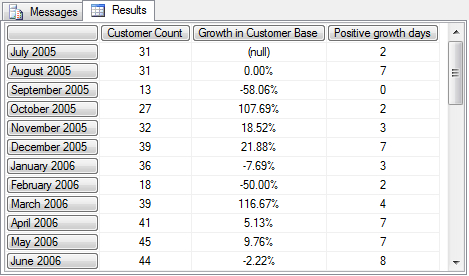### How it works…

The task says we need to count days for each time period and use only positive ones. Therefore, it might seem appropriate to perform iteration, which, in this case, can be performed using the `FILTER()` function.

But, there's a potential problem. We cannot expect to have days on rows, so we must use the `DESCENDANTS()` function to get all dates in the current context.

Finally, in order to get the number of items that came up upon filtering, we use the `COUNT` function.

### There's more…

Filter function is an iterative function which doesn't run in block mode, hence it will slow down the query. In the introduction, we said that it's always wise to search for an alternative if available. Let's see if something can be done here. A keen eye will notice a "count of filtered items" pattern in this expression. That pattern suggests the use of a set-based approach in the form of SUM-IF combination. The trick is to provide 1 for the True part of the condition taken from the `FILTER()` statement and null for the False part. The sum of one will be equivalent to the count of filtered items.

In other words, once rewritten, that same calculated member would look like this:

```MEMBER [Measures].[Positive growth days] AS
SUM(
Descendants([Date].[Fiscal].CurrentMember, , leaves),
IIF( [Measures].[Growth in Customer Base] > 0, 1, null)
)```

Execute the query using the new definition. Both the `SUM()` and the `IIF()` functions are optimized to run in the block mode, especially when one of the branches in `IIF()` is null. In this particular example, the impact on performance was not noticeable because the set of rows was relatively small. Applying this technique on large sets will result in drastic performance improvement as compared to the FILTER-COUNT approach. Be sure to remember that in future.

http://tinyurl.com/SumIIF

#### Hints for query improvements

There are several ways you can avoid the `FILTER()` function in order to improve performance.

• When you need to filter by non-numeric values (i.e. properties or other metadata), you should consider creating an attribute hierarchy for often-searched items and then do one of the following:

• Use a tuple when you need to get a value sliced by that new member

• Use the `EXCEPT()` function when you need to negate that member on its own hierarchy (NOT or <>)

• Use the `EXISTS()` function when you need to limit other hierarchies of the same dimension by that member

• Use the `NONEMPTY()` function when you need to operate on other dimensions, that is, subcubes created with that new member

• Use the 3-argument `EXISTS()` function instead of the `NONEMPTY()` function if you also want to get combinations with nulls in the corresponding measure group (nulls are available only when the NullProcessing property for a measure is set to `Preserve`)

• When you need to filter by values and then count a member in that set, you should consider aggregate functions like `SUM()` with `IIF()` part in its expression, as described earlier.

The next recipes, Iterating on a set in order to create a new one and Iterating on a set using recursion, deal with other methods of iteration.

## Iterating on a set in order to create a new one

There are situations when we don't want to eliminate certain members from a set, but instead execute a for-each type of loop. This is done using the GENERATE() function. In this recipe we'll show you how to create a new set of members from the existing one.

Start a new query in SSMS and check that you're working on the right database. Then write the following query:

```SELECT
NON EMPTY
{ [Date].[Calendar].[Calendar Year].MEMBERS *
[Measures].[Sales Amount] } ON 0,
NON EMPTY
{ [Sales Territory].[Sales Territory Country].MEMBERS }
ON 1
FROM

The query returns 4 years on columns and 6 countries on rows. This recipe shows how to get a set of best months, one for each year.

### How to do it…

Follow these steps to create a new set from the initial one:

1. Cut the years from columns and define a named set using them.

2. Name that set `Best month per year`.

3. Wrap that set in the `Generate()` function so that the set of years becomes its first argument.

4. The second argument should be the `TopCount()` function which uses the descendants of each year on the `Month` level and finds the best month according to the value of the measure `Sales Amount`.

5. Put the name of the new set on columns.

6. The final query should look as follows:

```WITH
SET [Best month per year] AS
Generate( [Date].[Calendar].[Calendar Year].MEMBERS,
TopCount(
Descendants( [Date].[Calendar].CurrentMember,
[Date].[Calendar].[Month],
SELF ),
1,
[Measures].[Sales Amount] )
)
SELECT
NON EMPTY
{ [Best month per year] *
[Measures].[Sales Amount] } ON 0,
NON EMPTY
{ [Sales Territory].[Sales Territory Country].MEMBERS } ON 1
FROM
7. Execute the query. Notice that each year is replaced with a single month, the month with the best sales result in that year: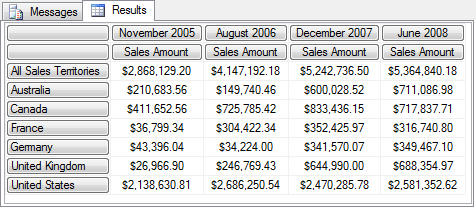### How it works…

The `Generate()` function can be thought of as a for-each loop. This means that we will iterate through each member of the initial set and assign another set instead of each member. That new set can have zero, one, or many members and this can vary during the iteration. In our example we're assigning a set with one member only, the best month in each year. That member is obtained using the `TopCount()` function where the first argument is months of the current year in iteration, the second argument is 1 (only one member to be returned), and the third argument is the `Sales Amount` measure — the criterion for deciding which month is the best. Months are obtained the standard way, using the `Descendants()` function.

### There's more…

The CURRENTORDINAL function is a special MDX function valid only in iterations. It returns the position of the current member (or tuple, to be precise) in the set in iteration (from 0 to N, where N is the total number of members in a set). In addition to that, there's also the CURRENT function. The `CURRENT` function returns the current tuple in a set being iterated. Again, it's only applicable during iterations.

Both of these functions can be used to detect the current tuple and to create various calculations with the current tuple and other tuples in that set. Reversing any initial set is one example of these manipulations. Comparing the value of the current tuple with the value of the previous tuple in the set (or any one before or after) in order to isolate certain tuples is another example.

Here's how you could reverse the set of months from the previous example.

```SET [Best month per year reversed] AS
Generate( [Date].[Calendar].[Calendar Year].MEMBERS
AS MySetAlias,
TopCount(
Descendants(
MySetAlias.Item( MySetAlias.Count –
MySetAlias.CurrentOrdinal
- 1 ).Item(0),
[Date].[Calendar].[Month],
SELF ),
1,
[Measures].[Sales Amount] )
)```

A set alias (`MySetAlias` in this example) is defined for the initial set. That set alias is later used for navigation. The combination of `Count` and `CurrentOrdinal` gives us members from the end of the set to its beginning, progressively, while the `Item()` function serves as a pointer on members in that set.

Yes, the same operation could be done simply by sorting the months by their member key, in descending order. Nevertheless, the idea of that example was to show you the principle which can be applied on any set, especially those that can't be reversed easily.

The other example mentioned above uses the `Filter()` function, not the `Generate()` function. There, tuples can be compared to each other progressively in order to see which one has the value higher than both of its neighboring members, which would signal that the current member is a relative peak. Or the opposite, whatever is more interesting in a particular case. However, the `Filter()` function doesn't add new members, it only limits its initial set and for that reason it is out of the scope of this recipe.

To summarize, `Current` () and `CurrentOrdinal ()` are powerful functions that allow us to perform self-joining type of operations in MDX or make use of the existing relations between dimensions and measure groups. These functions are useful not only in the `Generate()` function, but in other iterating functions as well, namely, the `Filter()` function.

#### Did you know

In MDX, there's no concept of the `FOR` loop. Iterations cannot be based on numbers (as in other languages or on other systems). They must always be based on a set. If we need to loop exactly N times, there are two basic ways we can achieve this. One is with the existing cube structure, the other is by expanding a cube with a utility dimension. The former means that we can use date dimension and take N members from its start. Or it could be some other dimension, as long as it has enough members to loop on. The latter, using the utility dimension, will be explained later in Chapter 8, Implementing the Tally table utility dimension

The recipes Iterating on a set using recursion and Iterating on a set in order to reduce it show other methods of iteration.

## Iterating on a set using recursion

Recursion is sometimes the best way to iterate a collection. Why? Because iterations using set functions (including the `GENERATE()` function) require that we loop through the whole set. But what if that set is big and we only need to find something in it? Wouldn't it be great to be able to stop the process when we've found what we wanted? Recursion enables just that – to stop when we're done.

In this recipe we're going to see how to calculate the average of an average using recursion.

To get started, start a new query in SSMS and check that you're working in the right database. Then write the following query:

```SELECT
{ [Measures].[Order Count] } ON 0,
NON EMPTY
{ Descendants( [Date].[Fiscal Weeks].[All Periods],
1 , SELF_AND_BEFORE) } ON 1
FROM

It returns 4 fiscal years and their total on top for the `Order Count` measure. Now let's see how to calculate the average daily value on the week level and the average weekly level on the year level, but based on the week level, not on the date level. In other words, each level will have the average value of members on the level immediately below.

### How to do it…

Follow these steps to perform recursion over a set:

1. Define a new calculated measure and name it `Average of an average`.

2. Use the `IIF()` function and specify its True parameter as the initial measure (`Order Count`).

3. The value should be returned for the leaf level, so the condition in `IIF()` should test exactly that using the `ISLEAF()` function.

4. In the False parameter we should provide the calculation we want to repeat recursively. In this case it is the `AVG()` function used on children of the current member.

5. The measure expression inside `AVG()` function should be the measure being defined.

6. Check if the measure is defined as follows:

```WITH
MEMBER [Measures].[Average of an average] AS
iif( IsLeaf( [Date].[Fiscal Weeks].CurrentMember ),
[Measures].[Order Count],
Avg( [Date].[Fiscal Weeks].CurrentMember.Children,
[Measures].[Average of an average] )
)
, FORMAT_STRING = '#,#'```
7. Don't forget to include that measure as the second measure on columns.

8. Run the query. The results will look as follows. The first row, the one with the All Periods member, will have the average yearly value as result, that is (56+84+435+195)/4=193. In turn, every year will have the average weekly value. The weekly values are not visible in this screenshot, but we can divide the Order Count values by 53, that is, by the number of weeks per year. That should give us the values for the Average of an average measure shown in the second column.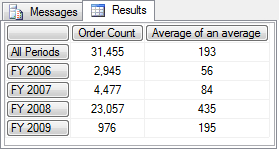### How it works…

Recursions are the most difficult iteration concept to apply. Their logic is very condensed. However, once you conquer them, you'll appreciate their power and efficiency. Let's see how that solution worked.

In order to start the recursive process, we have to specify an expression that uses the same calculated measure we're defining, thereby providing a different input parameter than the one which was being used in the current pass of recursive process. In order to stop the process, we must have a branch without the reference to that measure. On top of all that, we must perform some operation to collect values on the way. Complicated? Let's analyze our query.

Fiscal years on rows are not the leaf level of the Fiscal Weeks user hierarchy. Therefore, the expression inside the `IIF()` statement evaluates as False. This leads us to the part where we have to calculate the average value for each child of the current member. With a small detail, the calculation should be performed using the same measure we're evaluating!

The evaluation for the current year member cannot be completed and is therefore delayed until the calculation for all its child members (weeks in this case) is performed. One by one, each week of the year in context is passed inside the definition of this measure and evaluated.

In case of a leaf member, the Order Count measure would be evaluated and returned to the outer evaluation context. Otherwise, another turn of the child member's evaluation would occur. And so on until we would finally hit leaf-level members.

In this example, weeks are the leaf level of the hierarchy being used in the query. They would be evaluated using the True part of the condition. The True parameter is without reference to the measure we're calculating, which means the recursive path would be over. The value of the Order Count measure starting from the Week 1 of FY 2006 would be collected and saved in a temporary buffer. The same process would be repeated for all weeks of that year. Only then the average of them would be calculated and returned as a value for FY 2006. After which the process would repeat for subsequent years on rows.

Let's also mention that the value for the root member (All years) is calculated with the recursion depth of 2, meaning each year it is first evaluated as an average of its weeks and then the average of its years is calculated and returned as the final result.

### There's more…

You might be wondering how does one recognize when to use recursion and when to use other types of iteration? Look for some of these pointers: relative positions, relative granulation for calculation and stop logic. If there's a mention of going back or forth from the current member in a set, but there's no fixed span, then that might be a good lead to use recursion. If there's a relative stopping point, that's another sign. Finally, if there's no explicit requirement to loop through the whole set, but moreover a requirement to stop at some point in the process, that's a definite sign to try to apply recursion as a solution to the problem.

In case no such signs exist, it's perhaps better and easier to use simple types of iterations we covered in previous recipes. The other case when you should consider straightforward iteration is when the recursion would span over more than half of the members on a particular hierarchy, that pushes the SSAS engine into the slow cell-by-cell mode.

#### Earlier versions of SSAS

SSAS 2008 and later have better support for recursion than previous versions of SSAS. Optimizations have been added to the code in form of unlimited recursion depth. Versions prior to that may suffer from memory limitations in some extreme cases.

The recipes Iterating on a set in order to create a new one and Iterating on a set in order to reduce it illustrate other ways of iteration.

## Dissecting and debugging MDX queries

If you write a query involving complex calculations, you might have a hard time trying to debug it in, not if case there is a problem inside, but there is a way. By breaking complex sets and calculations into smaller ones and/or by converting those sets and members into strings, we can visually represent the intermediate results and thereby isolate the problematic part of the query.

True, there's no real debugger in the sense that you can pause the calculation process of the query and evaluate the variables. What you can do is to simulate that using this approach.

For this recipe we'll use the final query in the previous recipe, Iterating on a set using recursion. We have chosen this as our example because it's a relatively complex calculation and we want to check if we're doing the right thing.

### How to do it…

Follow these steps to create a calculated measure that shows the evaluation of another calculation:

1. Start SSMS and execute the following query:

```WITH
MEMBER [Measures].[Average of an average] AS
iif( IsLeaf( [Date].[Fiscal Weeks].CurrentMember ),
[Measures].[Order Count],
Avg( [Date].[Fiscal Weeks].CurrentMember.Children,
[Measures].[Average of an average] )
)
, FORMAT_STRING = '#,#'
SELECT
{ [Measures].[Order Count],
[Measures].[Average of an average] } ON 0,
NON EMPTY
{ Descendants( [Date].[Fiscal Weeks].[All Periods],
1 , SELF_AND_BEFORE) } ON 1
FROM
2. Create a new calculated measure and name it `Proof.`

3. Copy-paste to measure we're validating inside the definition of the `Average of an average` measure.

4. Leave the True part as is.

5. Modify the False part as shown in the next step below.

6. Finally, wrap the whole expression with one `IIF()` statement that checks whether the original measure is empty. The definition of that measure should look like this:

```MEMBER [Measures].[Proof] AS
iif( IsEmpty( [Measures].[Order Count] ),
null,
iif( IsLeaf( [Date].[Fiscal Weeks].CurrentMember ),
[Measures].[Order Count],
'( ' +
Generate( [Date].[Fiscal Weeks]
.CurrentMember.Children,
iif( IsEmpty( [Measures]
.[Average of an average] ),
'(null)',
CStr(
Round( [Measures]
.[Average of an average],
0 ) )
),
' + ' ) +
' ) / ' +
CStr( NonEmpty( [Date].[Fiscal Weeks]
.CurrentMember.Children,
[Measures].[Order Count]
).Count )
)
)```
7. Add that measure on columns and execute the query. The result will look like this: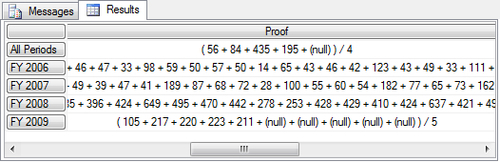### How it works…

The general principle of debugging MDX queries is to show some text, that is, current member names, their properties, position in a set, their descendants, and ancestors, whatever helps. Other times we'll convert the complete sets that we're operating with into a string, just to see the members inside and their order. For numeric values, if they are formed using several sub-calculations like in this example, we try to compose that evaluation as a string too. In short, we're displaying textual values of items we are interested in.

In our example, the main part where something interesting is going on is the False parameter of the inner `IIF`() function. Therefore, that's the place we're building a string in. Since it's relatively hard to explain what exactly we are doing there, let's take one more look at the previous image before we continue any further.

Measure `Proof` is a string representation of all individual values used to calculate each row. It is represented as a sum of N values, where N is number of children of the current member, divided by their count. Additionally, null values are preserved and displayed as well in the string, but the count omits them.

Now, the calculation itself. First, there's an open bracket in the form of a string. Then the `Generate()` function is applied, only this time it's the second version of that function, the one that returns not a set but a string. More information about it can be found here:

http://tinyurl.com/MDXGenerate

Partial strings generated during iteration need to be concatenated. For that reason the third argument of the `Generate()` function was used with the value "+".

The `Generate()` function, as explained in the recipe Iterating on a set in order to create a new one is a type of loop. In this case, it takes each child of a current member of the Fiscal Weeks user hierarchy and tests whether it is empty or not. If it is, a constant string is used (`'(null)'`), if not, the value of the measure is rounded to zero decimals.

Which measure? That same measure we're calculating the result for. Hence, it's again a call for iteration, this time using each child, one by one, because they are in the context at the time of call.

In the new pass, those members will be leaf members. They'll collect the value of measure `Order Count` and get out of that pass.

Once all the children are evaluated, the individual values will be concatenated using a " + " sign with a space on each side for better readability.

But the process is not over, only recursion is.

Next, we have to close the bracket which we opened in the beginning of the process and we have to calculate the denominator. Notice the measure inside the denominator is not calling for recursion. In order to get the count of members, we used the `NonEmpty()` function over the original measure. That returns the members which have values.

Finally, we haven't mentioned this specifically so far, but the outer `IIF()` statement checks if we're on a member that has no result. If so, we can skip that member. Remember, we had to do that because the inner part of the `Proof` measure is a string which is never null.

### There's more…

In the process of dissecting, evaluating and debugging calculations and queries, various MDX functions can be used. Some of them are mentioned below. However it is advised that you look for additional information on MSDN and other sources.

• String functions, namely MembertToStr and SetToStr , for converting members and sets into strings.

• Set functions, namely the Generate () function and especially its string variant, which is a very powerful method for iterating on a set and collecting partial calculations in form of strings.

• Metadata functions (also known as hierarchy and level functions), for collecting information about members and their hierarchies.

• Logical functions, for testing on leaf level and emptiness.

• VBA functions, for handling errors (IsError()) and string manipulations.

### Note

Don't forget to use the AddCalculatedMembers() function if you need to include calculated members.

#### Useful string functions

Here's a list of VBA functions that can be used in MDX:

http://tinyurl.com/MDXVBA

Here's a list of MDX functions grouped by types:

http://tinyurl.com/MDXfunctions

The recipe Optimizing MDX queries using NonEmpty() function shows how to keep only relevant members for debugging purposes and prevent all members of a hierarchy from being returned as the result.

## Using NON_EMPTY_BEHAVIOR

In SSAS 2005, the NON_EMPTY_BEHAVIOR property (NEB for short) was used for optimization of calculated members and cells as well as MDX script assignments. The optimization was done by indicating under which circumstances the expression can be ignored because the dependent expression returns null and vice versa. The rules for proper usage were either unknown or a bit too complex for many, so there was a lot of a misuse and incorrect results.

The situation changed with SSAS 2008. The new engine handled many scenarios automatically, without the NEB specified. It has been said that NEB is required only in a small number of situations where the expression is very complex or dense. In all others it should be left out.

The recipe in front of you shows how to specify NEB for calculated measure.

We're going to recycle the query in the recipe Dissecting and debugging MDX queries, the calculated member named `Proof`, to be precise, but we'll comment the NON EMPTY line of the query in order to see the effect of our action. Start a new query in SSMS and check that you're working on the right database, then write this query and execute it:

```WITH
MEMBER [Measures].[Average of an average] AS
iif( IsLeaf( [Date].[Fiscal Weeks].CurrentMember ),
[Measures].[Order Count],
Avg( [Date].[Fiscal Weeks].CurrentMember.Children,
[Measures].[Average of an average] )
)
, FORMAT_STRING = '#,#'
MEMBER [Measures].[Proof] AS
iif( IsEmpty( [Measures].[Order Count] ),
null,
iif( IsLeaf( [Date].[Fiscal Weeks].CurrentMember ),
[Measures].[Order Count],
'( ' +
Generate( [Date].[Fiscal Weeks]
.CurrentMember.Children,
iif( IsEmpty( [Measures]
.[Average of an average]),
'(null)',
CStr(
Round( [Measures]
.[Average of an average],
0 ) )
),
' + ' ) +
' ) / ' +
CStr( NonEmpty( [Date].[Fiscal Weeks]
.CurrentMember.Children,
[Measures].[Order Count]
).Count )
)
)
SELECT
{ [Measures].[Order Count],
[Measures].[Proof],
[Measures].[Average of an average] } ON 0,
//   NON EMPTY
{ Descendants( [Date].[Fiscal Weeks].[All Periods],
1 , SELF_AND_BEFORE) } ON 1
FROM

The idea is to provide the NEB expression for the `Proof` measure.

### How to do it…

1. Provide the NEB expression for the Proof measure and specify `[Measures].[Order Count]` for it. This is what the new part should look like:

`      , NON_EMPTY_BEHAVIOR = [Measures].[Order Count]`
2. Run the query again. Nothing has changed; the result is still the same and it was obtained as fast as the initial query.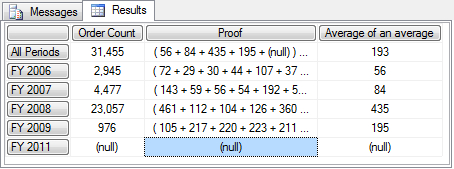### How it works…

As we said in the introduction to this recipe, SSAS engine handles most of the non-empty cells automatically and we don't have to provide NEB. In this experiment we noticed no visible improvement in query speed. Careful measurements using a tool called MDX Studio (http://tinyurl.com/MDXStudioFolder) revealed that NEB did two things: it caused fewer cells to be calculated (366 instead of 540), but it increased the duration of the query, although very, very little. In short, no benefit was gained from providing the NEB property.

### There's more…

The advantage of NEB manifests in complex calculations where many cells have to be calculated in order to get the result. NEB reduces that number of cells and hence makes such calculations faster.

In spite of its name, the true nature of the NON_EMPTY_BEHAVIOR property is that it is a performance hint only, not a function for removing empty cells. It's should be seen as an "empty values hint", not a "behavior". In other words, the NON_EMPTY_BEHAVIOR should not be used for removing empty cells instead of the IIF() function, NonEmpty() function or other similar functions just because that seems easier or more readable to do. True, in most cases it will work the same, but not always which makes it unreliable. Since it's only a hint and not a command like the previously mentioned functions, the engine might occasionally reject that suggestion and calculate on its own. Surely you don't want that to happen in your project. Therefore, experiment with NEB on complex calculations, having a hint might help the engine in that case. But if you need to remove empty cells, make sure there's also an adequate function for that inside the calculation, in addition to that hint.

#### Did you know?

NEB can be used in an MDX script as well as in a query.

NEB can contain not only one or more measures, but a tuple as well. More information about the NEB property and when and how it can be applied can be found in SSAS 2008 Performance Guide:

http://tinyurl.com/PerfGuide2008

The Optimizing MDX queries using the NonEmpty() function and Dissecting and debugging MDX queries recipes deal with reducing the number of rows returned from a query by identifying rows with null values.

## Optimizing MDX queries using the NonEmpty() function

NonEmpty() is a very powerful MDX function. It is primarily used to reduce sets in a very fast manner.

In the previous recipe we used it to get the count of children of the current member that are not empty for the measure Order Count. In this recipe, we'll show how it can be used again, this time using the Customer and Date dimensions.

Start a new query in SSMS and check that you're working on the right database. Then write the following query and execute it:

```SELECT
{ [Measures].[Internet Sales Amount] } ON 0,
NON EMPTY
Filter(
{ [Customer].[Customer].[Customer].MEMBERS } *
{ [Date].[Date].[Date].MEMBERS },
[Measures].[Internet Sales Amount] > 1000
) ON 1
FROM

The query shows the sales per customer and dates of their purchases and isolates only those combinations where the purchase was over 1000 USD.

After some time, maybe a minute or so, the query will return the results.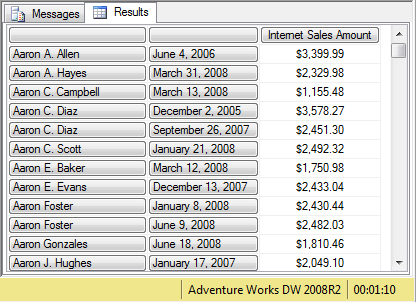Now let's see how to improve the execution time.

### How to do it…

Follow these steps to improve the query performance by adding the `NonEmpty()` function:

1. Wrap `NonEmpty()` around the cross-join of customers and dates so that it becomes the first argument of that function.

2. Use the measure on columns as the second argument of that function.

3. This is how the MDX query should look like:

```SELECT
{ [Measures].[Internet Sales Amount] } ON 0,
NON EMPTY
Filter(
NonEmpty(
{ [Customer].[Customer].[Customer].MEMBERS } *
{ [Date].[Date].[Date].MEMBERS },
{ [Measures].[Internet Sales Amount] }
),
[Measures].[Internet Sales Amount] > 1000
) ON 1
FROM
4. Execute that query and observe the results as well the time required for execution. The query returned the same results, only much faster, right?

### How it works…

Both the Customer and Date dimensions are medium-sized dimensions. The cross-join they make contains several million combinations. The `Filter()` operation is not optimized to work in block mode, which means a lot of calculations will have to be performed by the engine to evaluate the set on rows.

Fortunately, the `NonEmpty()` function exists. This function can be used to reduce any set, especially multidimensional sets that are result of a cross-join operation. A reduced set has fewer cells to be calculated and therefore the query runs much faster.

### There's more…

Regardless of the benefits that were shown in this recipe, `NonEmpty()` should be used with caution. The best practice says we should use it with sets (named sets and axes) as well as in functions which are not optimized to work in block mode, such as with the `Filter()` function. On the other hand, we should avoid using `NonEmpty()` in aggregate functions such as `Sum()` and other MDX set functions that are optimized to work in block mode. The use of `NonEmpty()` inside optimized functions will prevent them from evaluating the set in block mode. This is because the set will not be compact once it passes the `NonEmpty()` function. The function will break it into many small non-empty chunks and each of these chunks will have to be evaluated separately. This will inevitably increase the duration of the query. In such cases, it is better to leave the original set intact, no matter its size. The engine will know how to run over it in optimized mode.

#### Common mistakes and useful tips

If a second set in the `NonEmpty()` function is not provided, the expression is evaluated in the context of the current measure in the moment of evaluation and current members of attribute hierarchies, also in the time of evaluation. In other words, if you're defining a calculated measure and you forget to include a measure in the second set, the expression is evaluated for that same measure which leads to null, a default initial value of every measure. If you're simply evaluating the set on axis, it will be evaluated in the context of the current measure, the default measure in the cube or the one provided in the slicer. Again, perhaps not something you expected. In order to prevent these problems, ALWAYS include a measure in the second set.

`NonEmpty()` reduces sets, just like a few other functions, namely `Filter()` and `Existing()` do. But what's special about `NonEmpty()` is that it reduces sets extremely efficiently and quickly. Because of that, there are some rules about where to position `NonEmpty()` in calculations made by the composition of MDX functions (one function wrapping the other). If we're trying to detect multi-select, that is, multiple members in slicer, `NonEmpty()` should go inside with the `EXISTING` function/keyword outside. The reason is that although they both shrink sets efficiently, `NonEmpty()` works great if the set is intact. `EXISTING` is not affected by the order of members or compactness of the set. Therefore, `NonEmpty()` should be applied earlier.

You may get System.OutOfMemory errors if you use the `CrossJoin()` operation on many large hierarchies because the cross-join generates a cartesian product of those hierarchies. In that case, consider using `NonEmpty()` to reduce the space to a smaller subcube. Also, don't forget to group the hierarchies by their dimension inside the cross-join.

#### NonEmpty() versus NON EMPTY

Both the `NonEmpty()` function and the `NON EMPTY` keyword reduce sets, but they do it in a different way.

The `NON EMPTY` keyword removes empty rows, columns, or both, depending on the axis on which that keyword is used in the query. Therefore, the `NON EMPTY` operator tries to push the evaluation of cells to an early stage whenever possible. This way the set on axis comes already reduced and the final result is faster.

Take a look at the initial query in this recipe, remove the `Filter()` function, run the query, and notice how fast the results come although the multidimensional set again counts millions of tuples. The trick is that the `NON EMPTY` operator uses the set on the opposite axis, the columns, to reduce the set on rows. Therefore, it can be said that `NON EMPTY` is highly dependent on members on axes and their values in columns and rows.

Contrary to the `NON EMPTY` operator found only on axes, the `NonEmpty()` function can be used anywhere in the query.

The `NonEmpty()` function removes all the members from its first set where the value of one or more measures in the second set is empty. If no measure is specified, the function is evaluated in the context of the current member.

In other words, the `NonEmpty()` function is highly dependent on members in the second set, the slicer, or the current coordinate in general.

Using NON_EMPTY_BEHAVIOR.

## Implementing logical AND on members from the same hierarchy

This recipe shows how to implement AND logic using members from the same hierarchy. It is recommended that you read the recipe Implementing logical OR on members from different hierarchies before continuing with this recipe because you'll have a much better understanding of the problem and solution.

Start a new query in SSMS and check that you're working on the right database. Then type in the following query and execute it:

```SELECT
{ [Measures].[Reseller Order Quantity],
[Measures].[Reseller Order Count] } ON 0,
NON EMPTY
{ [Date].[Month of Year].MEMBERS } ON 1
FROM
WHERE
( [Promotion].[Promotion Type].&[New Product] )```

The query displays all months in the year with the New Product promotion type.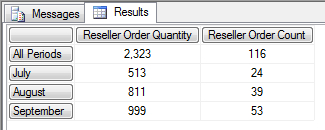If we replace the promotion in a slicer with another one, let's say Excess Inventory promotion type, we'll get one month less.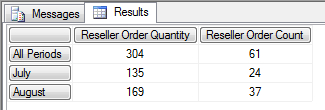The idea is to have a single query which displays the result where both of these promotion types occur in the same month. In other words, we want to show the values for July and August.

We have several ways of doing it, but this recipe will focus on the slicer-subselect solution. Other solutions will be mentioned in further sections of this recipe.

Let's take one closer look at possible combinations and their values in BIDS before we start.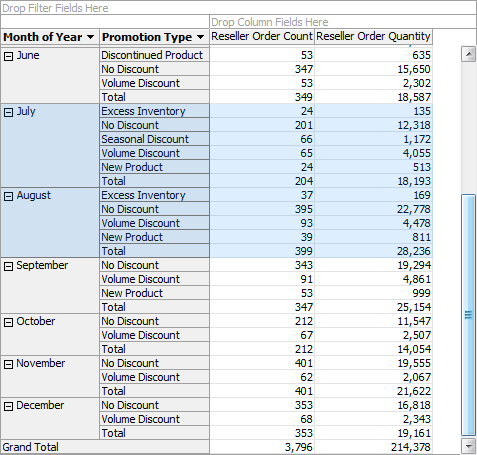### How to do it…

Follow these steps to implement the AND logic between members of the same hierarchy:

1. Rearrange the query so that it includes a subselect part with those two members inside (see the code that follows).

2. Put the Promotion Type hierarchy on rows instead of the Month of Year hierarchy.

3. Wrap the member in slicer (member of Promotion Type hierarchy) with the `EXISTS()` function so that it becomes its second argument.

4. Put the Month of Year level (not hierarchy!) as the first argument. Level has 3-part syntax; hierarchy has 2-part syntax. Don't omit the third part because it won't work.

5. Provide the measure group of interest, in this case it is "Reseller Sales".

6. Wrap another `EXISTS()` function around the previous one so that the previous becomes its first argument.

7. Add the second member of the Promotion Type hierarchy as the second argument of that function. Use the same measure group as before.

8. Run the following query:

```SELECT
{ [Measures].[Reseller Order Quantity],
[Measures].[Reseller Order Count] } ON 0,
NON EMPTY
{ [Promotion].[Promotion Type].MEMBERS } ON 1
FROM
(
SELECT
{ [Promotion].[Promotion Type].&[Excess Inventory],
[Promotion].[Promotion Type].&[New Product] } ON 0
FROM
)
WHERE
(
Exists(
Exists( { [Date].[Month of Year].[Month of Year].MEMBERS },
{ [Promotion].[Promotion Type].&[Excess Inventory] },
"Reseller Sales"
),
{ [Promotion].[Promotion Type].&[New Product] },
"Reseller Sales"
)
)```
9. The result of the query shows the aggregate for July and August, months where both promotion types occur: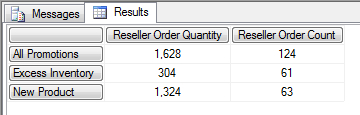10. Compare these results with the ones in the previous image (showing combination of promotion types and months) and you'll notice the aggregates match the sum of individual values.

### How it works…

In order to perform AND logic on the same hierarchy, we must cascade the conditions. The inner set returns all the months that have the Excess Inventory promotion type (that's 3 of them as seen on the initial screenshot). The outer set restricts the inner set even more by filtering out all months which don't have the other promotion type as well. That leaves only two months, July and August.

Since there is no MDX expression that would work and return the AND result using those two members only, we must use another granularity as the base of the report. In this case, months that survive the combined restriction are months for which the result will be returned.

It is totally up to us to decide which hierarchy and which level we want to use as a new granularity as long as we adhere to some common sense rules.

First, the relation between new hierarchy's level members and the members for slicing should be many-to-many. This is always so in case of different dimensions (Promotion and Date), a case covered in this example. In case of the same dimension, the solution will work only for a single member that is related to both members in the AND operation. Whether that will be something other than the `All` member depends on the hierarchy and members selected for the AND operation. For example, two promotion types used in this example share only one ancestor – the `All` member, which can be verified in the Promotions user hierarchy of that dimension.

Second, the whole idea has to be valid. If we have two promotions and they occur on order items, then it's fine to use orders for granularity in slicer. However, if we have two resellers and they occur on orders, then this time it is not OK to use orders because there will never be an intersection of two resellers on the same order. We must use something else, a higher granularity like a day, promotion, or similar where two resellers can exist together. That's the basic idea.

Which hierarchy to use? That usually becomes quite obvious once we ask ourselves the question behind the report. For example, the last query, as seen in the previous screenshot, returned the two promotion types we started with this recipe, promotion types that come together on a monthly basis. Two things are important here: together and basis. The term "together" represents the AND logic. The term "monthly basis" is in fact the new granularity for the report (that which goes in slicer).

That explains the slicer part of the solution. What about the subselect part? Why is it there?

The subselect part serves the purpose of adjusting the results. Without it, we would get the wrong total. Let me explain this in more detail.

If you remove the subselect part of the query and execute it again, it will return the result displayed in the following image.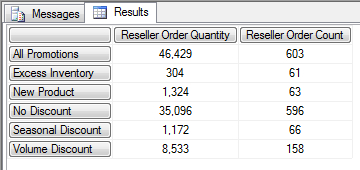The numbers on this image match the aggregated values displayed in one of the previous images that show the combination of promotion types and months, the highlighted part of it. Those months were now in the slicer; hence the result is aggregated by them (603 orders = 204 in July + 399 in August and so on for every cell).

The query returned all promotion types because nothing limited them in the query. The slicer effectively has months, not promotion types, right?

There are two things we can do to correct it, that is, to display the result for those two hierarchies only. One is to put them in slicer so that they cross-join with the existing slicer. The other is to put them in subselect. I prefer the second option because that way we can still have them on an axis of the query. Otherwise we have a conflict with the slicer (a hierarchy cannot appear in slicer and on an axis, but it can appear in subselect and on an axis). That's why the subselect was used.

The subselect, as seen before, limits the promotion types that appear on an axis and adjusts their total so that is becomes the visual total for those two members. This is exactly what we need, the value for individual promotion types and their correct total.

To conclude, in order to implement AND logic, we have done two things. First, we have established a new granularity in slicer. Second, we used the subselect to adjust the total.

### There's more…

This is not the only way to implement AND logic. We can do it on axis as well. In that case all we have to do is put a construct from the slicer on rows and leave the subselect as is.

#### Where to put what?

Based on a request, the AND logic can be implemented on rows or in slicer. If there's a request to hide the hierarchy for which we're applying the AND logic, we should put the corresponding MDX expression in slicer. On the other hand, if there's an explicit request to show members on rows, we must put the construct on rows. There we can cross-join it with additional hierarchies if required.

#### A very complex scenario

In case of a more complex scenario where 3 different hierarchies need to be combined, we can apply the same solution, which in a general case should have N cascades in slicer and N members in subselect. The N is the number of members from the same hierarchy.

In case we need to combine many members using AND logic, some of them originating from different hierarchies and some from the same, the solution becomes very complex.

You are advised to watch for the order in cascades and dimensionality of the potential tuples.

A recipe with similar theme is Implementing logical OR on members from different hierarchies.